Скачать презентацию Introduction to Software Testing Chapter 4 Input Space

ea0809dff83ab49f7275db07b62e060c.ppt

• Количество слайдов: 32Introduction to Software Testing Chapter 4 Input Space Partition Testing Paul Ammann & Jeff Offutt www. introsoftwaretesting. com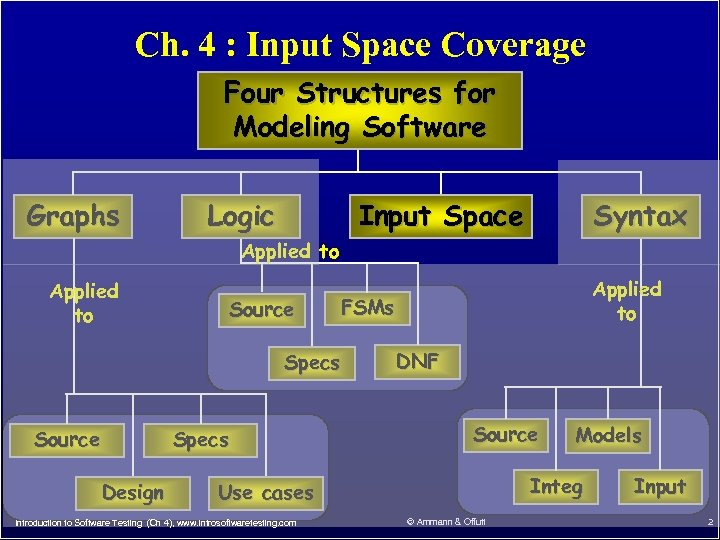Ch. 4 : Input Space Coverage Four Structures for Modeling Software Graphs Logic Input Space Syntax Applied to Source FSMs Specs Source Specs Design Applied to DNF Source Integ Use cases Introduction to Software Testing (Ch 4), www. introsoftwaretesting. com Models © Ammann & Offutt Input 2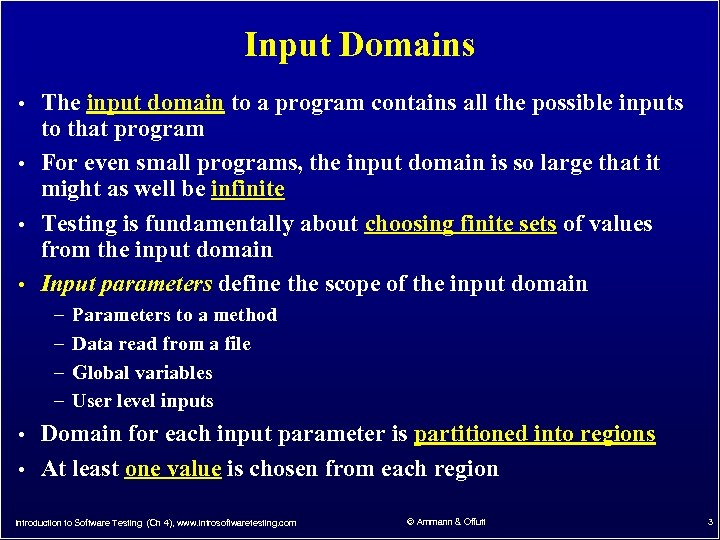Input Domains • The input domain to a program contains all the possible inputs to that program • For even small programs, the input domain is so large that it might as well be infinite • Testing is fundamentally about choosing finite sets of values from the input domain • Input parameters define the scope of the input domain – – Parameters to a method Data read from a file Global variables User level inputs • Domain for each input parameter is partitioned into regions • At least one value is chosen from each region Introduction to Software Testing (Ch 4), www. introsoftwaretesting. com © Ammann & Offutt 3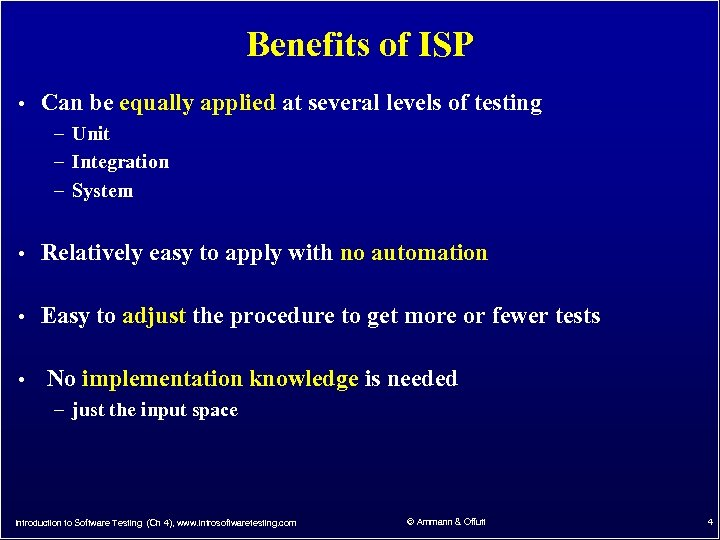Benefits of ISP • Can be equally applied at several levels of testing – Unit – Integration – System • Relatively easy to apply with no automation • Easy to adjust the procedure to get more or fewer tests • No implementation knowledge is needed – just the input space Introduction to Software Testing (Ch 4), www. introsoftwaretesting. com © Ammann & Offutt 4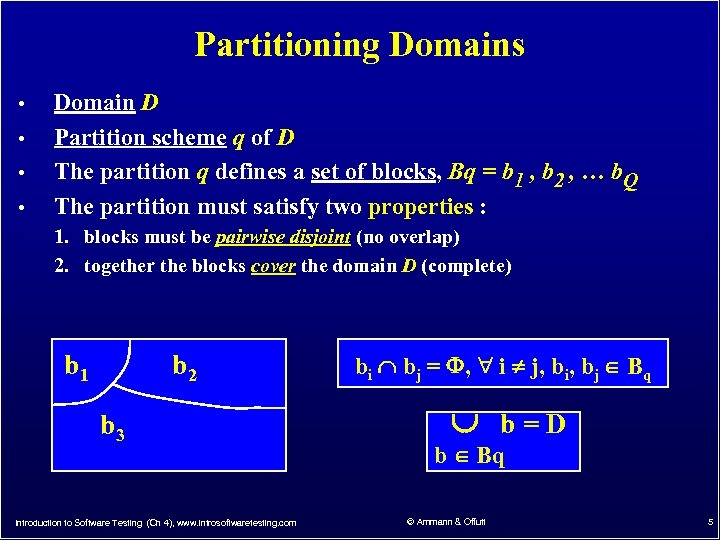Partitioning Domains • • Domain D Partition scheme q of D The partition q defines a set of blocks, Bq = b 1 , b 2 , … b. Q The partition must satisfy two properties : 1. blocks must be pairwise disjoint (no overlap) 2. together the blocks cover the domain D (complete) b 1 b 2 b 3 Introduction to Software Testing (Ch 4), www. introsoftwaretesting. com bi bj = , i j, bi, bj Bq b=D b Bq © Ammann & Offutt 5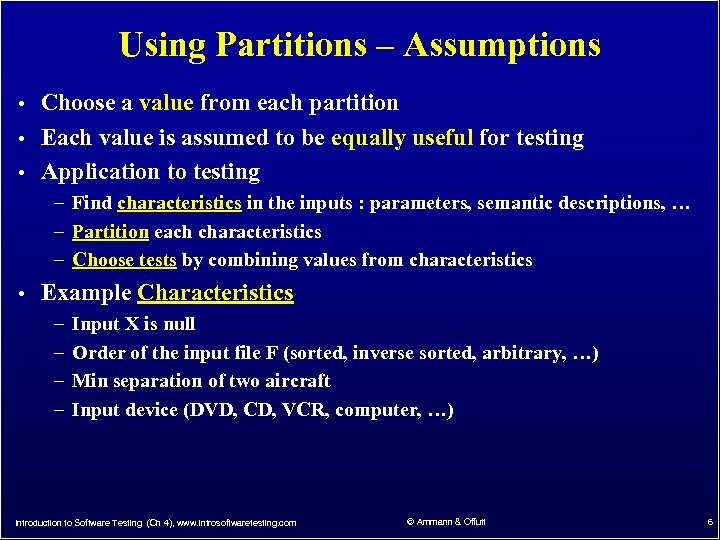Using Partitions – Assumptions • Choose a value from each partition • Each value is assumed to be equally useful for testing • Application to testing – Find characteristics in the inputs : parameters, semantic descriptions, … – Partition each characteristics – Choose tests by combining values from characteristics • Example Characteristics – Input X is null – Order of the input file F (sorted, inverse sorted, arbitrary, …) – Min separation of two aircraft – Input device (DVD, CD, VCR, computer, …) Introduction to Software Testing (Ch 4), www. introsoftwaretesting. com © Ammann & Offutt 6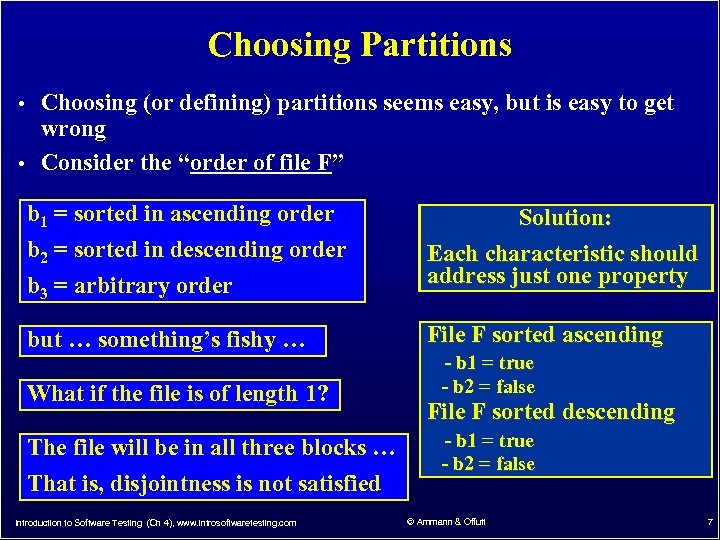Choosing Partitions • Choosing (or defining) partitions seems easy, but is easy to get wrong • Consider the “order of file F” b 1 = sorted in ascending order b 2 = sorted in descending order b 3 = arbitrary order but … something’s fishy … What if the file is of length 1? The file will be in all three blocks … That is, disjointness is not satisfied Introduction to Software Testing (Ch 4), www. introsoftwaretesting. com Solution: Each characteristic should address just one property File F sorted ascending - b 1 = true - b 2 = false File F sorted descending - b 1 = true - b 2 = false © Ammann & Offutt 7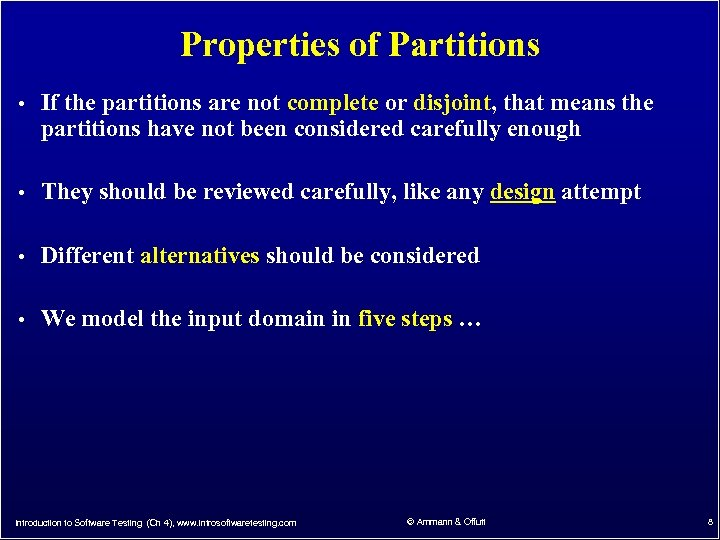Properties of Partitions • If the partitions are not complete or disjoint, that means the partitions have not been considered carefully enough • They should be reviewed carefully, like any design attempt • Different alternatives should be considered • We model the input domain in five steps … Introduction to Software Testing (Ch 4), www. introsoftwaretesting. com © Ammann & Offutt 8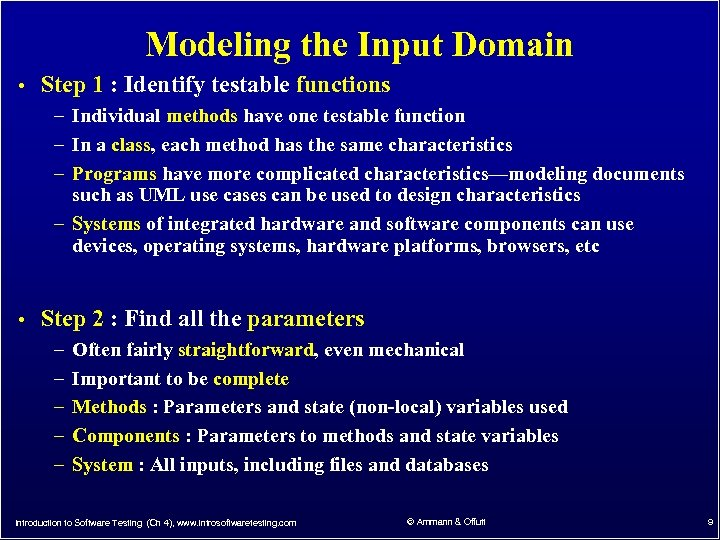Modeling the Input Domain • Step 1 : Identify testable functions – Individual methods have one testable function – In a class, each method has the same characteristics – Programs have more complicated characteristics—modeling documents such as UML use cases can be used to design characteristics – Systems of integrated hardware and software components can use devices, operating systems, hardware platforms, browsers, etc • Step 2 : Find all the parameters – Often fairly straightforward, even mechanical – Important to be complete – Methods : Parameters and state (non-local) variables used – Components : Parameters to methods and state variables – System : All inputs, including files and databases Introduction to Software Testing (Ch 4), www. introsoftwaretesting. com © Ammann & Offutt 9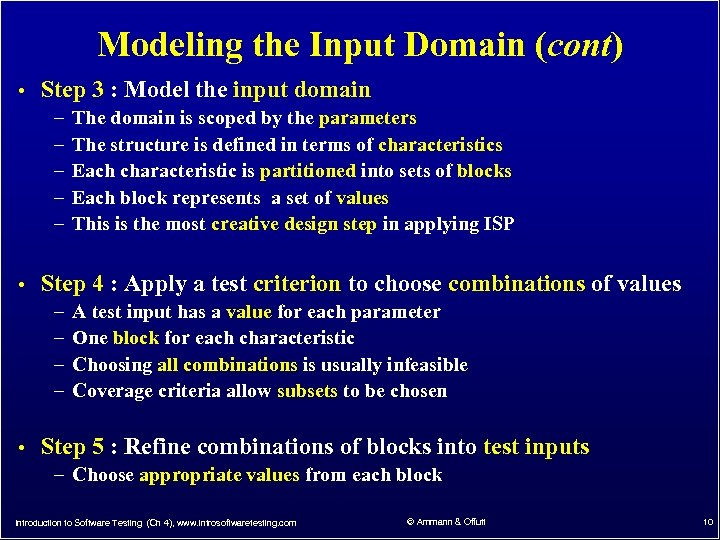Modeling the Input Domain (cont) • Step 3 : Model the input domain – The domain is scoped by the parameters – The structure is defined in terms of characteristics – Each characteristic is partitioned into sets of blocks – Each block represents a set of values – This is the most creative design step in applying ISP • Step 4 : Apply a test criterion to choose combinations of values – A test input has a value for each parameter – One block for each characteristic – Choosing all combinations is usually infeasible – Coverage criteria allow subsets to be chosen • Step 5 : Refine combinations of blocks into test inputs – Choose appropriate values from each block Introduction to Software Testing (Ch 4), www. introsoftwaretesting. com © Ammann & Offutt 10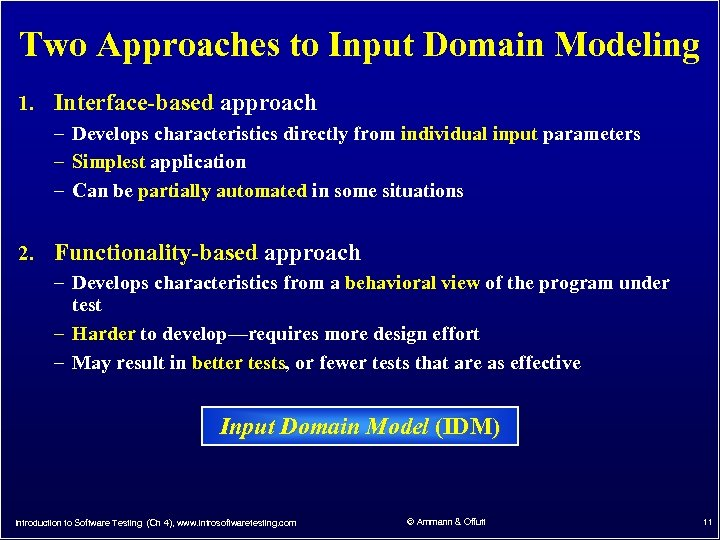Two Approaches to Input Domain Modeling 1. Interface-based approach – Develops characteristics directly from individual input parameters – Simplest application – Can be partially automated in some situations 2. Functionality-based approach – Develops characteristics from a behavioral view of the program under test – Harder to develop—requires more design effort – May result in better tests, or fewer tests that are as effective Input Domain Model (IDM) Introduction to Software Testing (Ch 4), www. introsoftwaretesting. com © Ammann & Offutt 11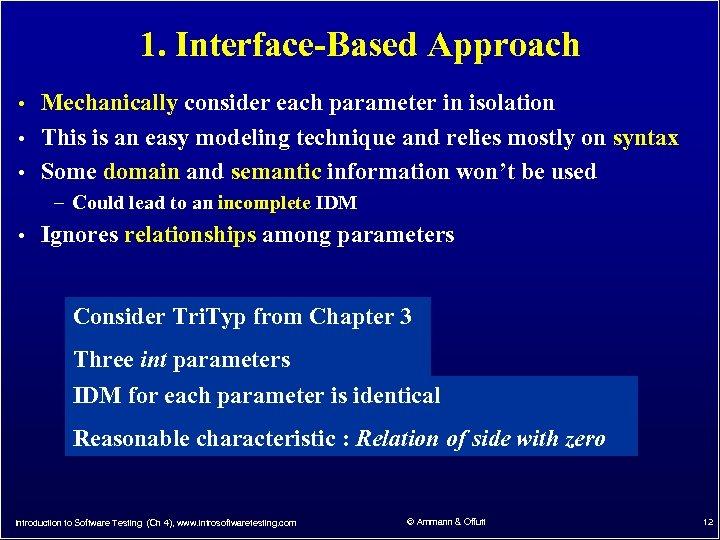1. Interface-Based Approach • Mechanically consider each parameter in isolation • This is an easy modeling technique and relies mostly on syntax • Some domain and semantic information won’t be used – Could lead to an incomplete IDM • Ignores relationships among parameters Consider Tri. Typ from Chapter 3 Three int parameters IDM for each parameter is identical Reasonable characteristic : Relation of side with zero Introduction to Software Testing (Ch 4), www. introsoftwaretesting. com © Ammann & Offutt 12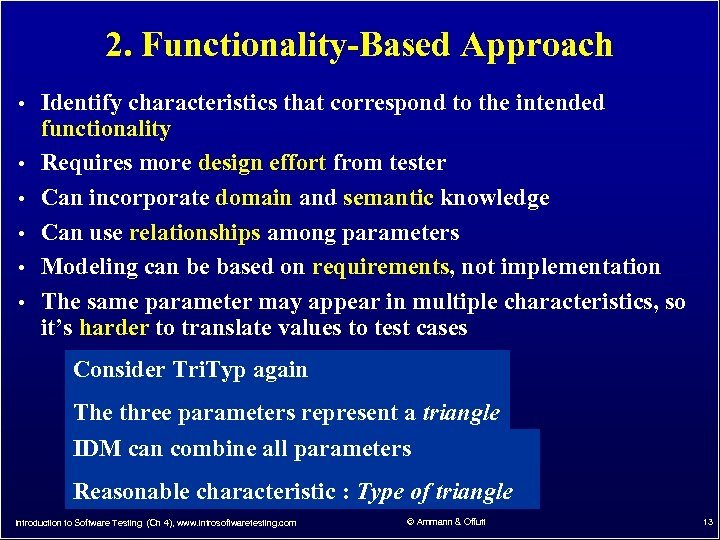2. Functionality-Based Approach • Identify characteristics that correspond to the intended • • • functionality Requires more design effort from tester Can incorporate domain and semantic knowledge Can use relationships among parameters Modeling can be based on requirements, not implementation The same parameter may appear in multiple characteristics, so it’s harder to translate values to test cases Consider Tri. Typ again The three parameters represent a triangle IDM can combine all parameters Reasonable characteristic : Type of triangle Introduction to Software Testing (Ch 4), www. introsoftwaretesting. com © Ammann & Offutt 13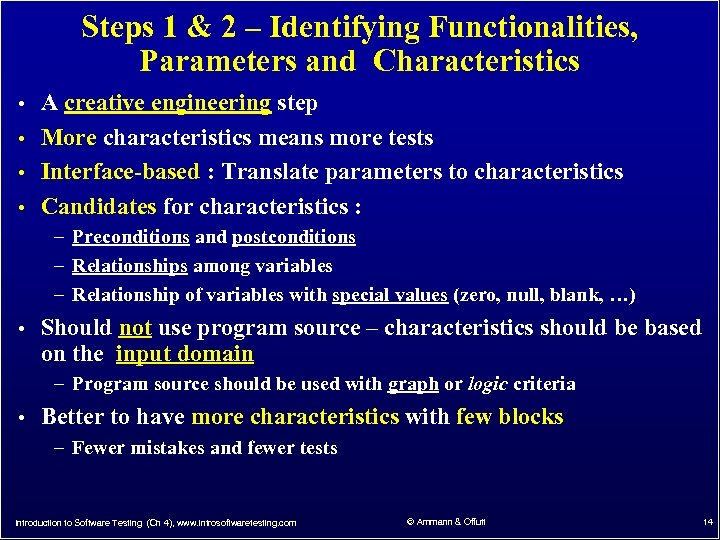Steps 1 & 2 – Identifying Functionalities, Parameters and Characteristics • A creative engineering step • More characteristics means more tests • Interface-based : Translate parameters to characteristics • Candidates for characteristics : – Preconditions and postconditions – Relationships among variables – Relationship of variables with special values (zero, null, blank, …) • Should not use program source – characteristics should be based on the input domain – Program source should be used with graph or logic criteria • Better to have more characteristics with few blocks – Fewer mistakes and fewer tests Introduction to Software Testing (Ch 4), www. introsoftwaretesting. com © Ammann & Offutt 14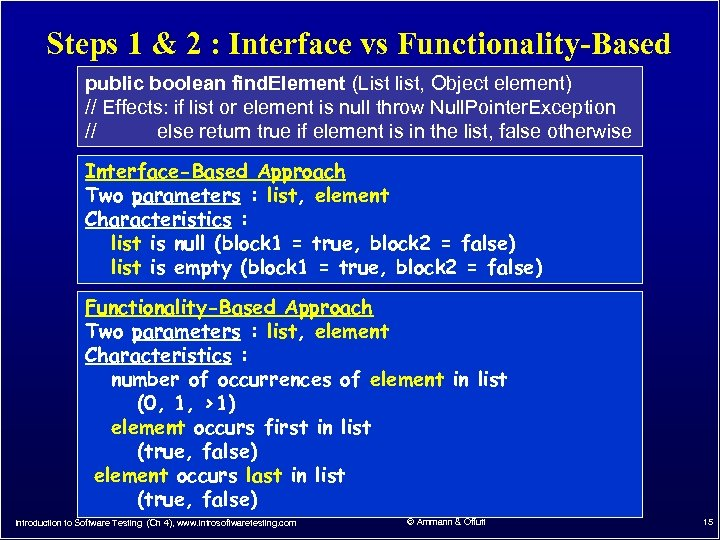Steps 1 & 2 : Interface vs Functionality-Based public boolean find. Element (List list, Object element) // Effects: if list or element is null throw Null. Pointer. Exception // else return true if element is in the list, false otherwise Interface-Based Approach Two parameters : list, element Characteristics : list is null (block 1 = true, block 2 = false) list is empty (block 1 = true, block 2 = false) Functionality-Based Approach Two parameters : list, element Characteristics : number of occurrences of element in list (0, 1, >1) element occurs first in list (true, false) element occurs last in list (true, false) Introduction to Software Testing (Ch 4), www. introsoftwaretesting. com © Ammann & Offutt 15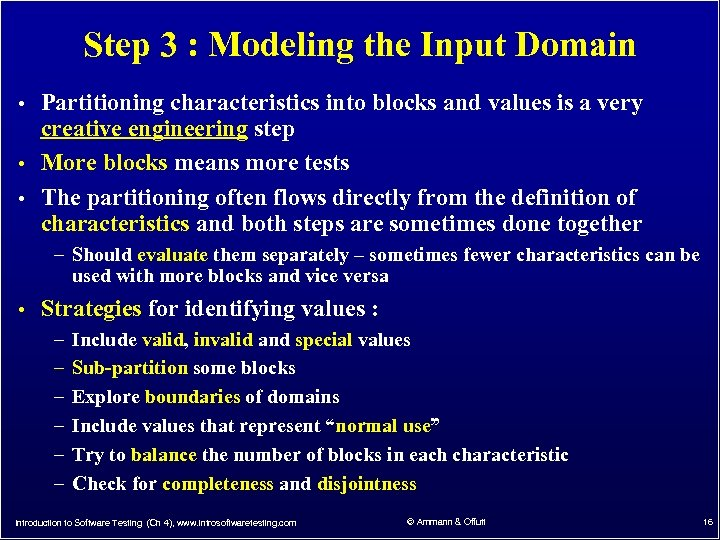Step 3 : Modeling the Input Domain • Partitioning characteristics into blocks and values is a very creative engineering step • More blocks means more tests • The partitioning often flows directly from the definition of characteristics and both steps are sometimes done together – Should evaluate them separately – sometimes fewer characteristics can be used with more blocks and vice versa • Strategies for identifying values : – Include valid, invalid and special values – Sub-partition some blocks – Explore boundaries of domains – Include values that represent “normal use” – Try to balance the number of blocks in each characteristic – Check for completeness and disjointness Introduction to Software Testing (Ch 4), www. introsoftwaretesting. com © Ammann & Offutt 16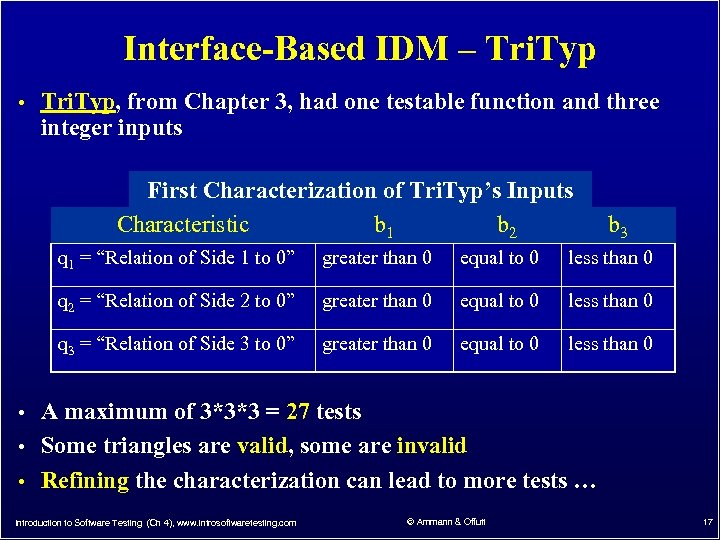Interface-Based IDM – Tri. Typ • Tri. Typ, from Chapter 3, had one testable function and three integer inputs First Characterization of Tri. Typ’s Inputs Characteristic b 1 b 2 b 3 q 1 = “Relation of Side 1 to 0” greater than 0 equal to 0 less than 0 q 2 = “Relation of Side 2 to 0” greater than 0 equal to 0 less than 0 q 3 = “Relation of Side 3 to 0” greater than 0 equal to 0 less than 0 • A maximum of 3*3*3 = 27 tests • Some triangles are valid, some are invalid • Refining the characterization can lead to more tests … Introduction to Software Testing (Ch 4), www. introsoftwaretesting. com © Ammann & Offutt 17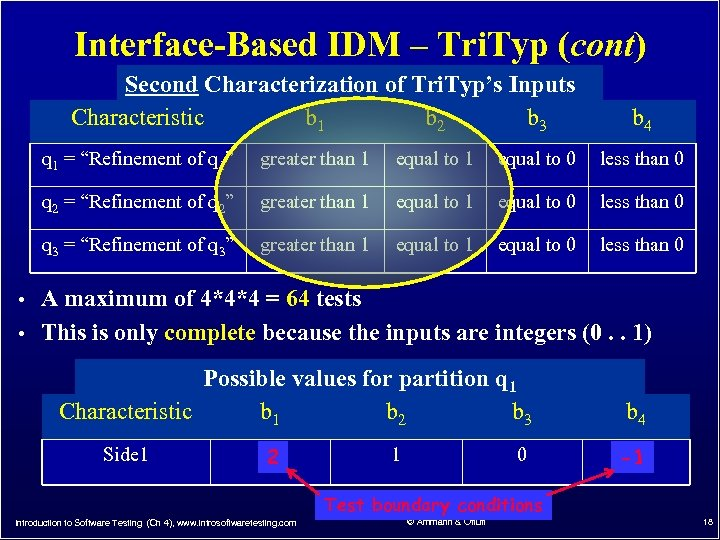Interface-Based IDM – Tri. Typ (cont) Second Characterization of Tri. Typ’s Inputs Characteristic b 1 b 2 b 3 b 4 q 1 = “Refinement of q 1” greater than 1 equal to 0 less than 0 q 2 = “Refinement of q 2” greater than 1 equal to 0 less than 0 q 3 = “Refinement of q 3” greater than 1 equal to 0 less than 0 • A maximum of 4*4*4 = 64 tests • This is only complete because the inputs are integers (0. . 1) Possible values for partition q 1 Characteristic b 1 b 2 b 3 Side 1 5 2 1 0 b 4 -5 -1 Test boundary conditions Introduction to Software Testing (Ch 4), www. introsoftwaretesting. com © Ammann & Offutt 18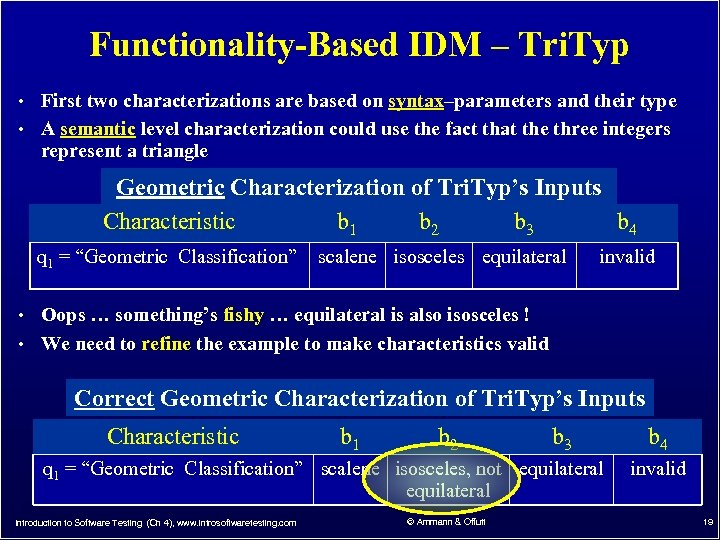Functionality-Based IDM – Tri. Typ • First two characterizations are based on syntax–parameters and their type • A semantic level characterization could use the fact that the three integers represent a triangle Geometric Characterization of Tri. Typ’s Inputs Characteristic b 1 b 2 b 3 b 4 q 1 = “Geometric Classification” scalene isosceles equilateral invalid • Oops … something’s fishy … equilateral is also isosceles ! • We need to refine the example to make characteristics valid Correct Geometric Characterization of Tri. Typ’s Inputs Characteristic b 1 b 2 b 3 q 1 = “Geometric Classification” scalene isosceles, not equilateral Introduction to Software Testing (Ch 4), www. introsoftwaretesting. com © Ammann & Offutt b 4 invalid 19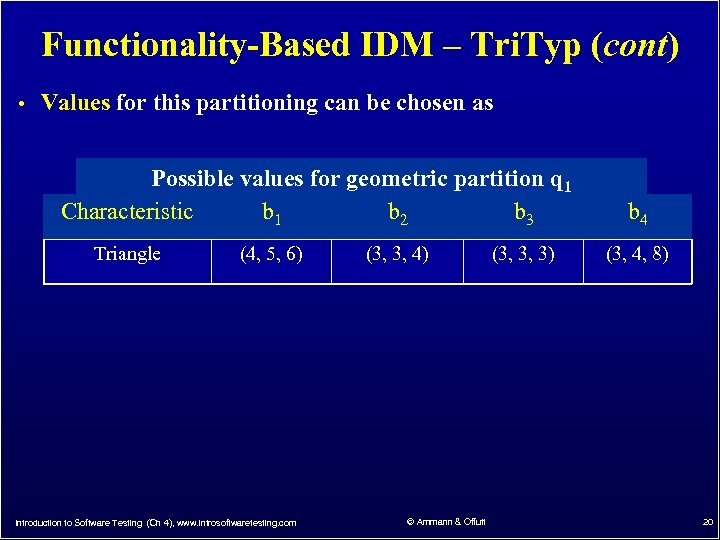Functionality-Based IDM – Tri. Typ (cont) • Values for this partitioning can be chosen as Possible values for geometric partition q 1 Characteristic b 1 b 2 b 3 Triangle (4, 5, 6) Introduction to Software Testing (Ch 4), www. introsoftwaretesting. com (3, 3, 4) © Ammann & Offutt (3, 3, 3) b 4 (3, 4, 8) 20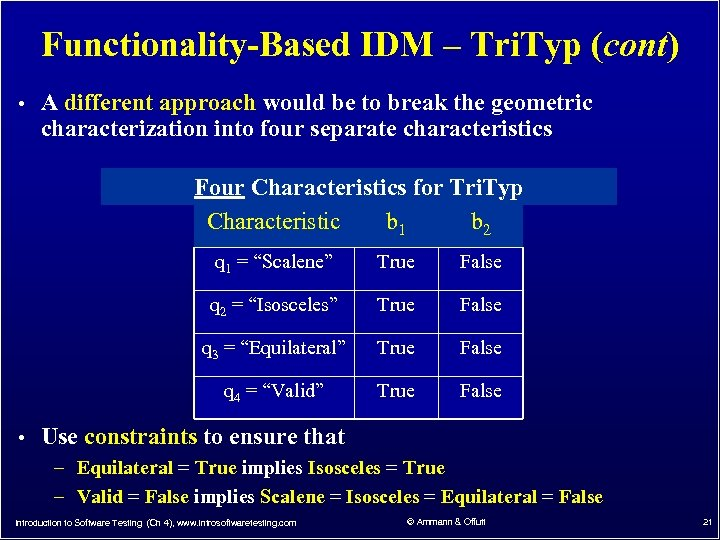Functionality-Based IDM – Tri. Typ (cont) • A different approach would be to break the geometric characterization into four separate characteristics Four Characteristics for Tri. Typ Characteristic b 1 b 2 q 1 = “Scalene” True False q 2 = “Isosceles” True False q 3 = “Equilateral” True False q 4 = “Valid” True False • Use constraints to ensure that – Equilateral = True implies Isosceles = True – Valid = False implies Scalene = Isosceles = Equilateral = False Introduction to Software Testing (Ch 4), www. introsoftwaretesting. com © Ammann & Offutt 21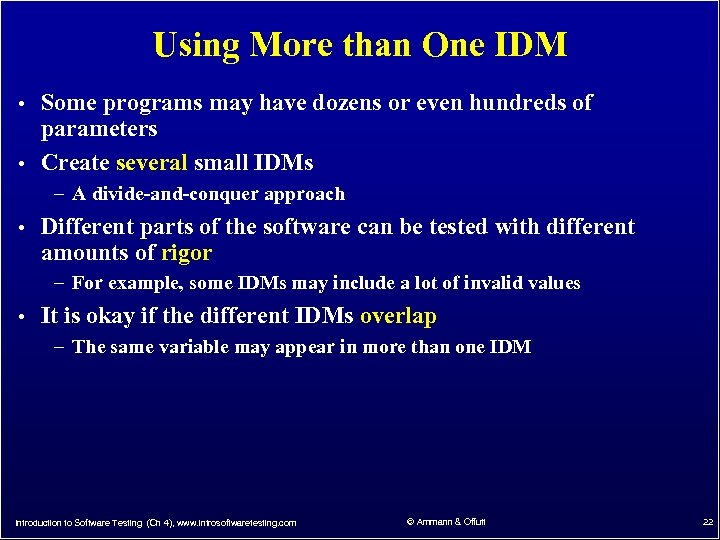Using More than One IDM • Some programs may have dozens or even hundreds of parameters • Create several small IDMs – A divide-and-conquer approach • Different parts of the software can be tested with different amounts of rigor – For example, some IDMs may include a lot of invalid values • It is okay if the different IDMs overlap – The same variable may appear in more than one IDM Introduction to Software Testing (Ch 4), www. introsoftwaretesting. com © Ammann & Offutt 22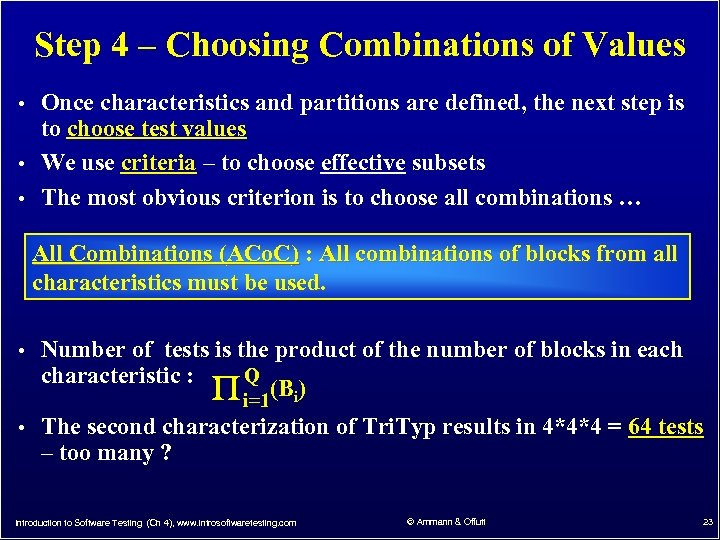Step 4 – Choosing Combinations of Values • Once characteristics and partitions are defined, the next step is to choose test values • We use criteria – to choose effective subsets • The most obvious criterion is to choose all combinations … All Combinations (ACo. C) : All combinations of blocks from all characteristics must be used. • Number of tests is the product of the number of blocks in each Q characteristic : (B ) i=1 i • The second characterization of Tri. Typ results in 4*4*4 = 64 tests – too many ? Introduction to Software Testing (Ch 4), www. introsoftwaretesting. com © Ammann & Offutt 23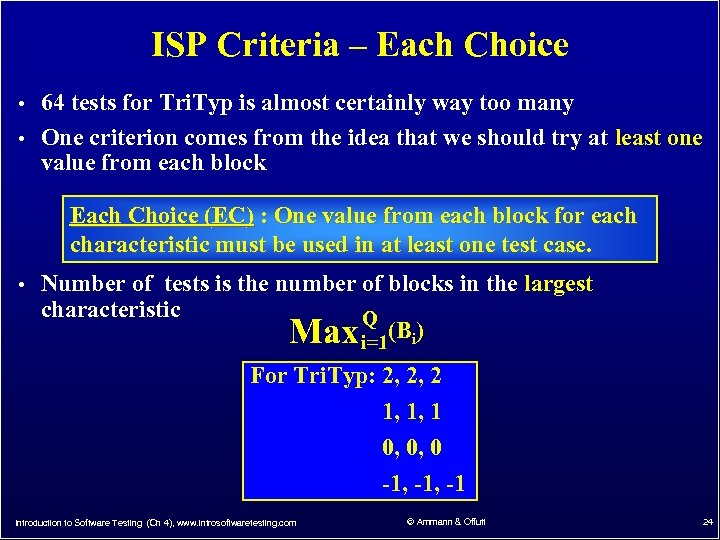ISP Criteria – Each Choice • 64 tests for Tri. Typ is almost certainly way too many • One criterion comes from the idea that we should try at least one value from each block Each Choice (EC) : One value from each block for each characteristic must be used in at least one test case. • Number of tests is the number of blocks in the largest characteristic Max Q (B ) i=1 i For Tri. Typ: 2, 2, 2 1, 1, 1 0, 0, 0 -1, -1 Introduction to Software Testing (Ch 4), www. introsoftwaretesting. com © Ammann & Offutt 24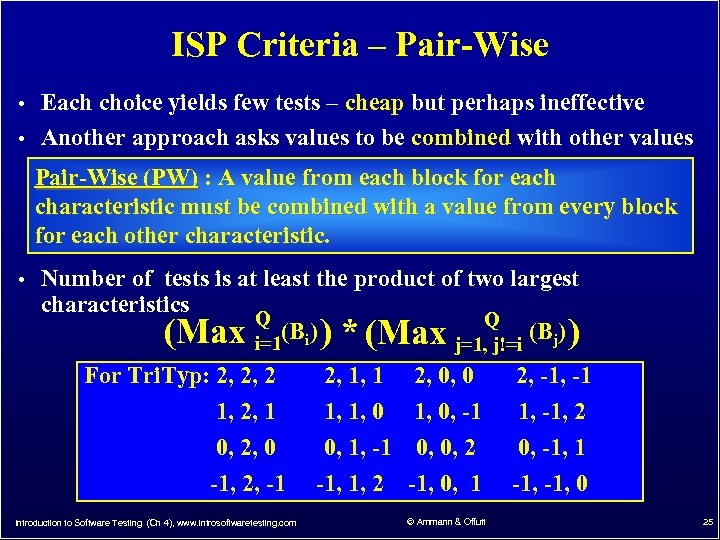ISP Criteria – Pair-Wise • Each choice yields few tests – cheap but perhaps ineffective • Another approach asks values to be combined with other values Pair-Wise (PW) : A value from each block for each characteristic must be combined with a value from every block for each other characteristic. • Number of tests is at least the product of two largest characteristics (Max Q (B ) i=1 i ) * (Max Q j=1, j!=i (Bj) ) For Tri. Typ: 2, 2, 2 2, 1, 1 2, 0, 0 2, -1 1, 2, 1 1, 1, 0, -1 1, -1, 2 0, 1, -1 0, 0, 2 -1, 1, 2 -1, 0, 1 0, -1, 1 -1, 0 0, 2, 0 -1, 2, -1 Introduction to Software Testing (Ch 4), www. introsoftwaretesting. com © Ammann & Offutt 25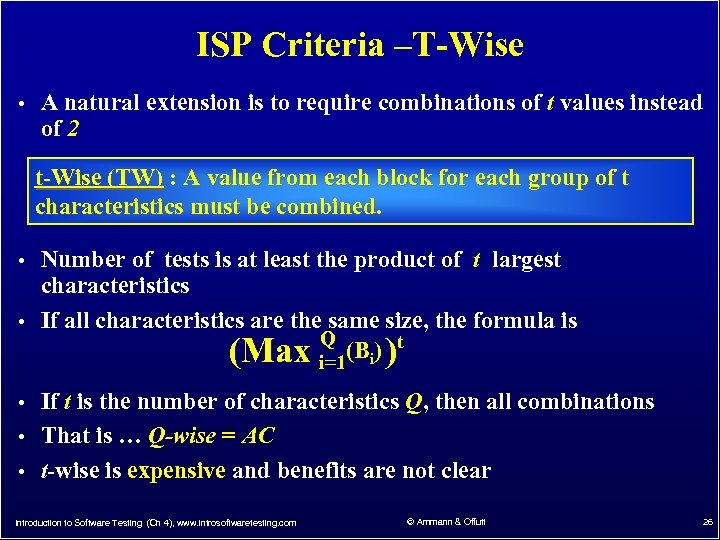ISP Criteria –T-Wise • A natural extension is to require combinations of t values instead of 2 t-Wise (TW) : A value from each block for each group of t characteristics must be combined. • Number of tests is at least the product of t largest characteristics • If all characteristics are the same size, the formula is Q (Bi) )t (Max i=1 • If t is the number of characteristics Q, then all combinations • That is … Q-wise = AC • t-wise is expensive and benefits are not clear Introduction to Software Testing (Ch 4), www. introsoftwaretesting. com © Ammann & Offutt 26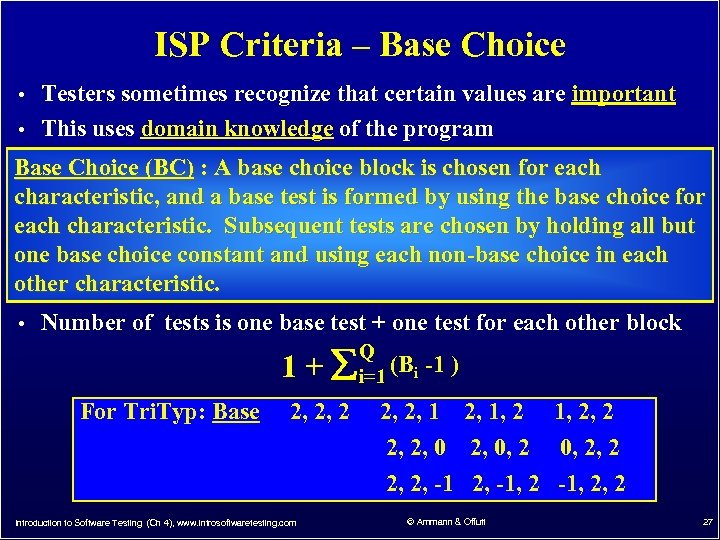ISP Criteria – Base Choice • Testers sometimes recognize that certain values are important • This uses domain knowledge of the program Base Choice (BC) : A base choice block is chosen for each characteristic, and a base test is formed by using the base choice for each characteristic. Subsequent tests are chosen by holding all but one base choice constant and using each non-base choice in each other characteristic. • Number of tests is one base test + one test for each other block Q 1 + i=1 (Bi -1 ) For Tri. Typ: Base 2, 2, 2, 1, 2, 2, 0, 2, 2, -1, 2, 2 Introduction to Software Testing (Ch 4), www. introsoftwaretesting. com © Ammann & Offutt 27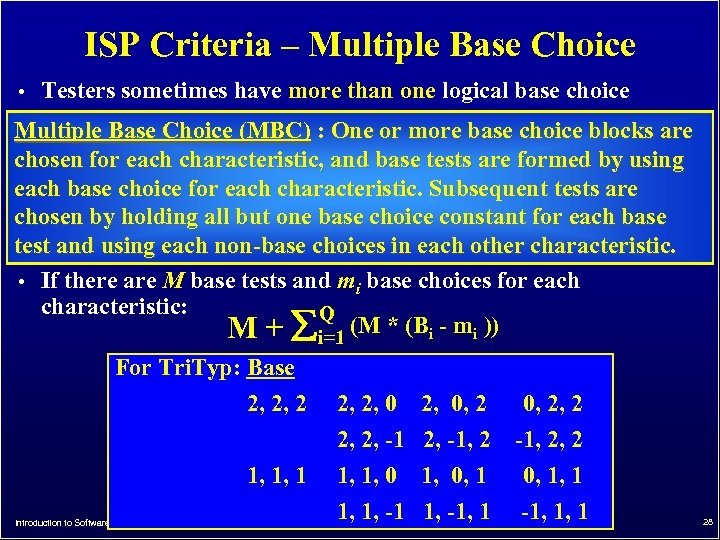ISP Criteria – Multiple Base Choice • Testers sometimes have more than one logical base choice Multiple Base Choice (MBC) : One or more base choice blocks are chosen for each characteristic, and base tests are formed by using each base choice for each characteristic. Subsequent tests are chosen by holding all but one base choice constant for each base test and using each non-base choices in each other characteristic. • If there are M base tests and mi base choices for each characteristic: Q M + i=1 (M * (Bi - mi )) For Tri. Typ: Base 2, 2, 2, 0, 2, 2 1, 1, 1 2, 2, -1, 2 1, 1, 0, 1 1, 1, -1, 1 -1, 2, 2 0, 1, 1 -1, 1, 1 Introduction to Software Testing (Ch 4), www. introsoftwaretesting. com © Ammann & Offutt 28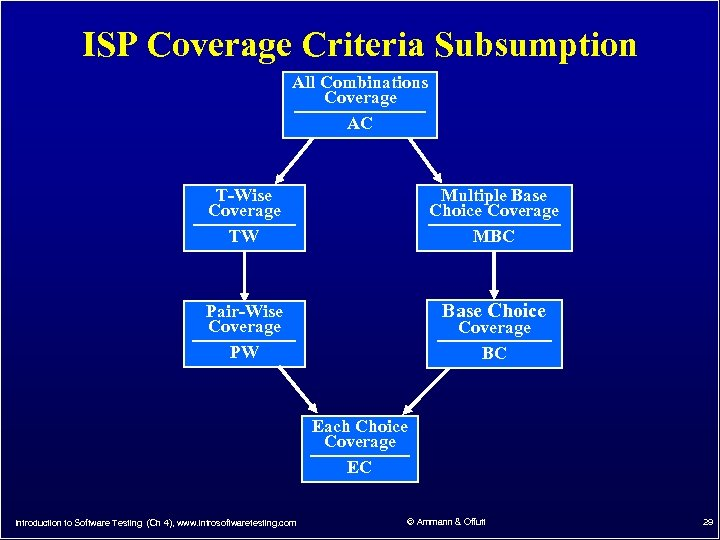ISP Coverage Criteria Subsumption All Combinations Coverage AC T-Wise Coverage TW Multiple Base Choice Coverage MBC Pair-Wise Coverage PW Base Choice Coverage BC Each Choice Coverage EC Introduction to Software Testing (Ch 4), www. introsoftwaretesting. com © Ammann & Offutt 29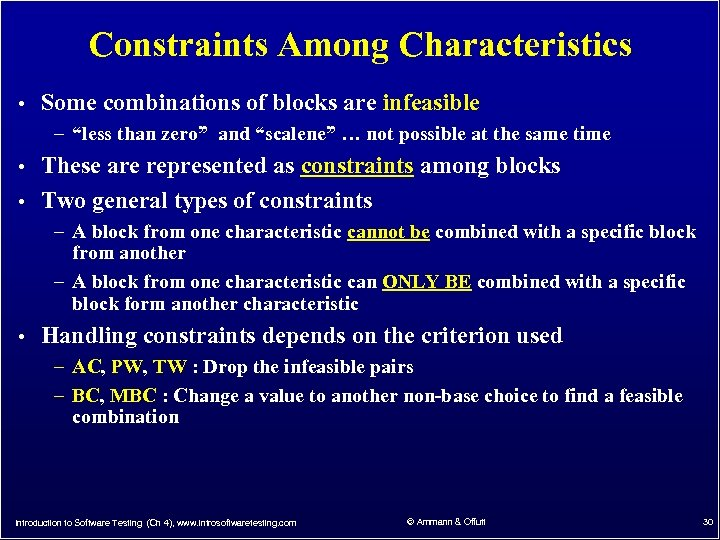Constraints Among Characteristics • Some combinations of blocks are infeasible – “less than zero” and “scalene” … not possible at the same time • These are represented as constraints among blocks • Two general types of constraints – A block from one characteristic cannot be combined with a specific block from another – A block from one characteristic can ONLY BE combined with a specific block form another characteristic • Handling constraints depends on the criterion used – AC, PW, TW : Drop the infeasible pairs – BC, MBC : Change a value to another non-base choice to find a feasible combination Introduction to Software Testing (Ch 4), www. introsoftwaretesting. com © Ammann & Offutt 30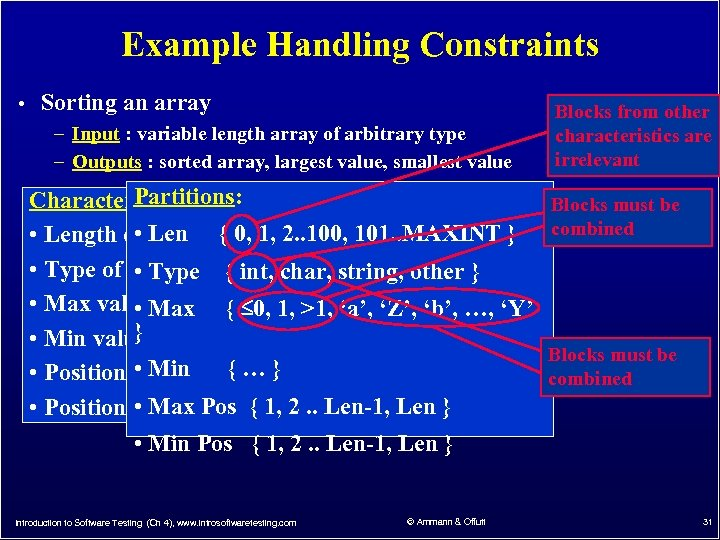Example Handling Constraints • Sorting an array – Input : variable length array of arbitrary type – Outputs : sorted array, largest value, smallest value Partitions: Characteristics: • array • Length of Len { 0, 1, 2. . 100, 101. . MAXINT } • Type of elements { int, char, string, other } • Type • Max value. Max { 0, 1, >1, ‘a’, ‘Z’, ‘b’, …, ‘Y’ • } • Min value • max { … • Position of. Min value } • min value • Position of. Max Pos { 1, 2. . Len-1, Len } Blocks from other characteristics are irrelevant Blocks must be combined • Min Pos { 1, 2. . Len-1, Len } Introduction to Software Testing (Ch 4), www. introsoftwaretesting. com © Ammann & Offutt 31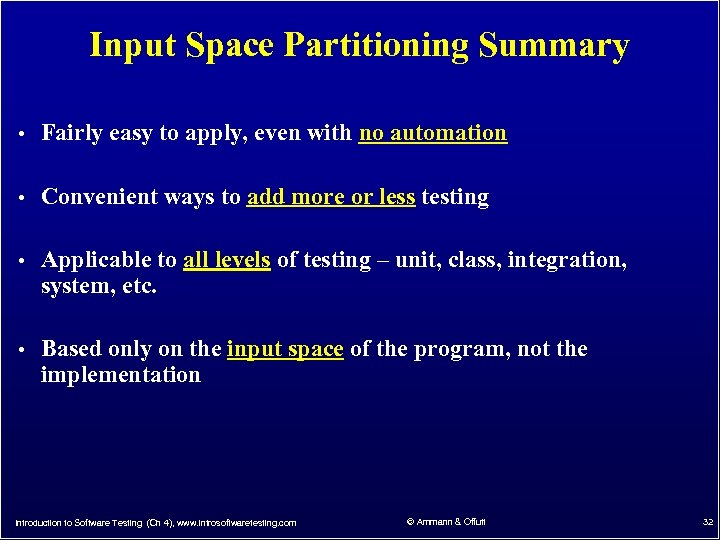Input Space Partitioning Summary • Fairly easy to apply, even with no automation • Convenient ways to add more or less testing • Applicable to all levels of testing – unit, class, integration, system, etc. • Based only on the input space of the program, not the implementation Introduction to Software Testing (Ch 4), www. introsoftwaretesting. com © Ammann & Offutt 32